# Atomic Structure and Periodic TrendsPage 4

#### WATCH ALL SLIDES

n = 1, 2, 3, 4, .

l = 0, 1, 2, 3, (n-1)

ml = -l, -l+1, -l+2, 0, ., l-1, l

Important

Slide 29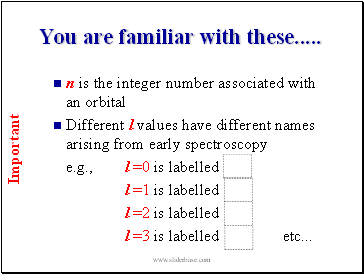You are familiar with these .

n is the integer number associated with an orbital

Different l values have different names arising from early spectroscopy

e.g., l =0 is labelled

l =1 is labelled

l =2 is labelled

l =3 is labelled etc .

Important

Slide 30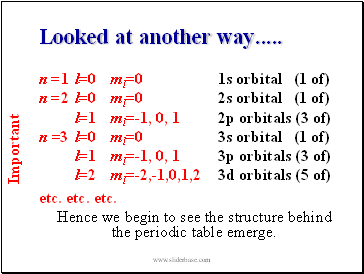Looked at another way .

n =1 l=0 ml=0 1s orbital (1 of)

n =2 l=0 ml=0 2s orbital (1 of)

l=1 ml=-1, 0, 1 2p orbitals (3 of)

n =3 l=0 ml=0 3s orbital (1 of)

l=1 ml=-1, 0, 1 3p orbitals (3 of)

l=2 ml=-2,-1,0,1,2 3d orbitals (5 of)

etc. etc. etc.

Hence we begin to see the structure behind the periodic table emerge.

Important

Slide 31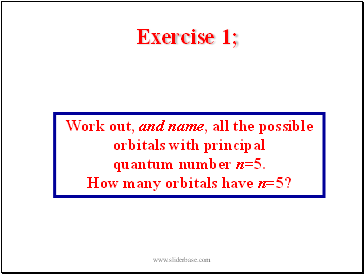Exercise 1;

Work out, and name, all the possible

orbitals with principal

quantum number n=5.

How many orbitals have n=5?

Slide 32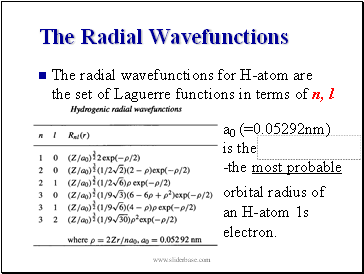The radial wavefunctions for H-atom are the set of Laguerre functions in terms of n, l

a0 (=0.05292nm)

is the

-the most probable

orbital radius of an H-atom 1s electron.

Slide 33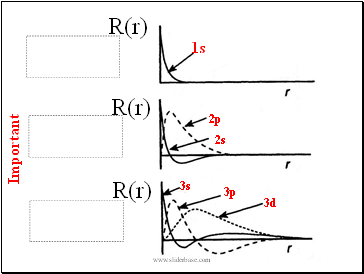R(r)

1s

2s

2p

3s

3p

3d

Important

R(r)

R(r)

Slide 34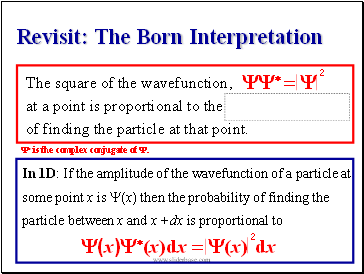## Revisit: The Born Interpretation

The square of the wavefunction,

at a point is proportional to the

of finding the particle at that point.

Y* = Y

In 1D: If the amplitude of the wavefunction of a particle at some point x is Y(x) then the probability of finding the particle between x and x +dx is proportional to

(x)Y*(x)dx = (x)

Y* is the complex conjugate of Y.

2

2

dx

Slide 35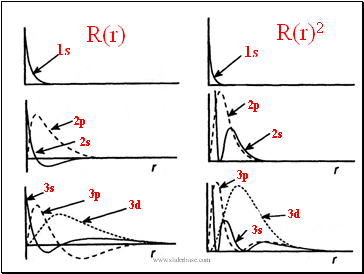R(r)2

R(r)

1s

2s

2p

3s

3d

1s

2s

2p

3s

3p

3d

3p

Slide 36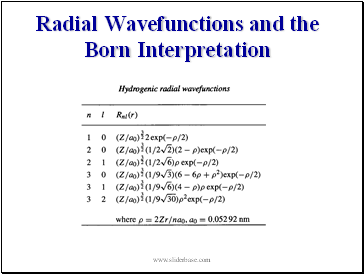## Radial Wavefunctions and the Born Interpretation

Slide 37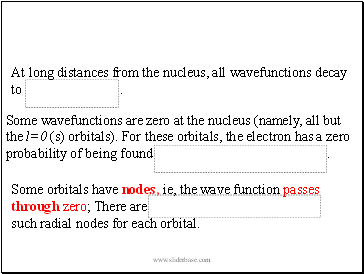At long distances from the nucleus, all wavefunctions decay to .

Some wavefunctions are zero at the nucleus (namely, all but the l=0 (s) orbitals). For these orbitals, the electron has a zero probability of being found .

Some orbitals have nodes, ie, the wave function passes through zero; There are such radial nodes for each orbital.

Go to page:
1  2  3  4  5  6  7  8  9  10  11  12  13  14  15
16  17